Courses

# Phase Equilibria (Part - 1) - Thermodynamic and Statistical Physics, CSIR-NET Physical Sciences Physics Notes | EduRev

## Physics for IIT JAM, UGC - NET, CSIR NET

Created by: Akhilesh Thakur

## Physics : Phase Equilibria (Part - 1) - Thermodynamic and Statistical Physics, CSIR-NET Physical Sciences Physics Notes | EduRev

The document Phase Equilibria (Part - 1) - Thermodynamic and Statistical Physics, CSIR-NET Physical Sciences Physics Notes | EduRev is a part of the Physics Course Physics for IIT JAM, UGC - NET, CSIR NET.
All you need of Physics at this link: Physics

Phase Equilibrium

We are all familiar with phase transitions where a substance transforms from one stable phase to another at an equilibrium temperature. For example, ice will be in equilibrium with liquid water at 273.15 K and 1 atmosphere pressure, or liquid water will be in equilibrium with water vapor at 373.15 K and one atmosphere. We will now take a look at the thermodynamic principles involved in phase transitions.

The only things we know are the criteria for equilibrium under certain conditions. That is, we know that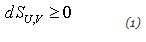for a closed isolated system (that is, the entropy seeks a maximum), and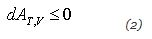for a system as constant temperature and volume (that is,the Helmholtz free energy seeks a minimum), and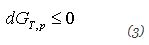for a system at constant temperature and pressure (the Gibbs free energy seeks a minimum).

We will do a series of three thought experiments under different sets of conditions and use the above criteria to tell us things about the temperature, pressure, and chemical potential of a system of two phases in equilibrium.

I. A closed isolated system.

Consider a closed isolated system consisting of two phases, phase α in equilibrium with phase β . We will call the temperature of the α phase Tα and the temperature of the β phase Tβ.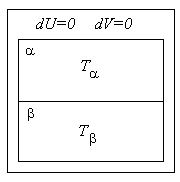We do not know, yet, what is the relationship between Tα and Tβ. We do know that in this system entropy seeks a maximum. That is,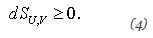Let us transfer a small amount of heat, dq, reversibly from phase α to phase β . For definiteness we will set dq > 0. So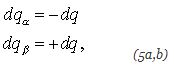and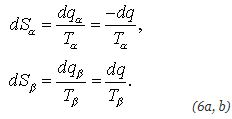The total entropy change for the system is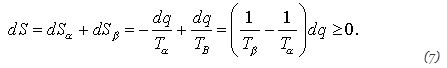Since dq is positive by construction we conclude that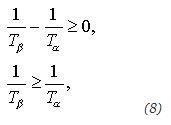or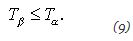If the system is not at equilibrium then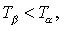which makes sense because heat flows spontaneously from a higher temperature to a lower temperature.

If the system is at equilibrium then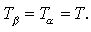There is only one temperature defined and there in no need to distinguish between the temperatures of the two phases.

159 docs

,

,

,

,

,

,

,

,

,

,

,

,

,

,

,

,

,

,

,

,

,

,

,

,

;